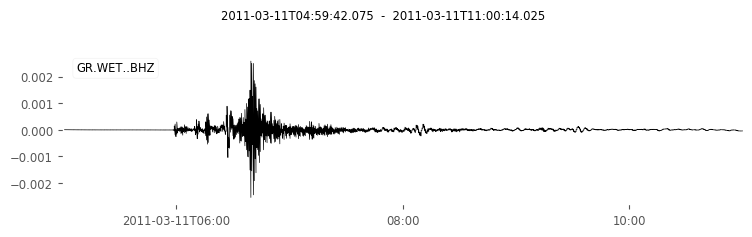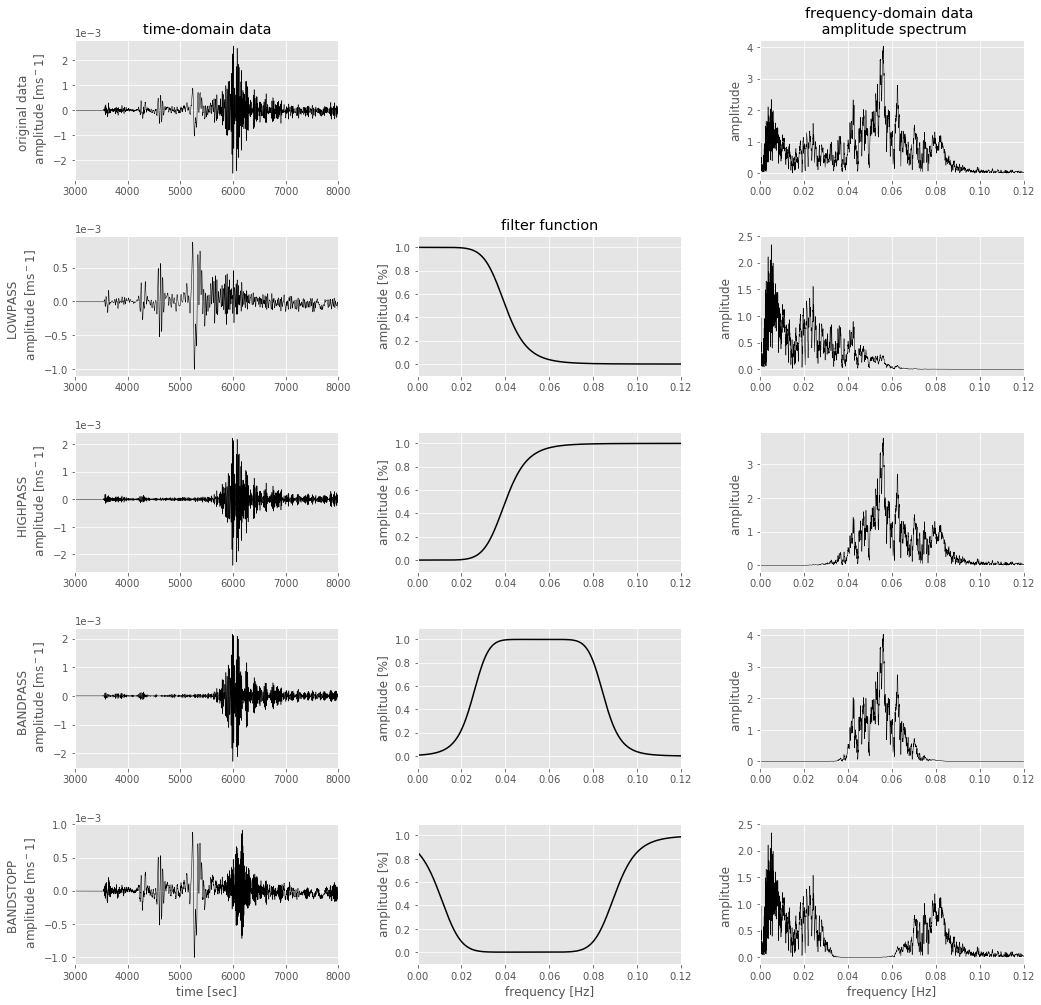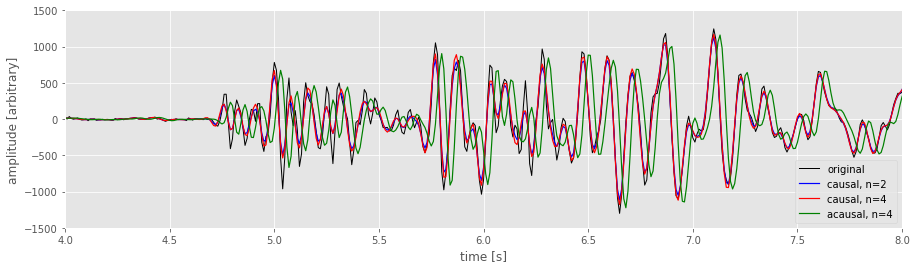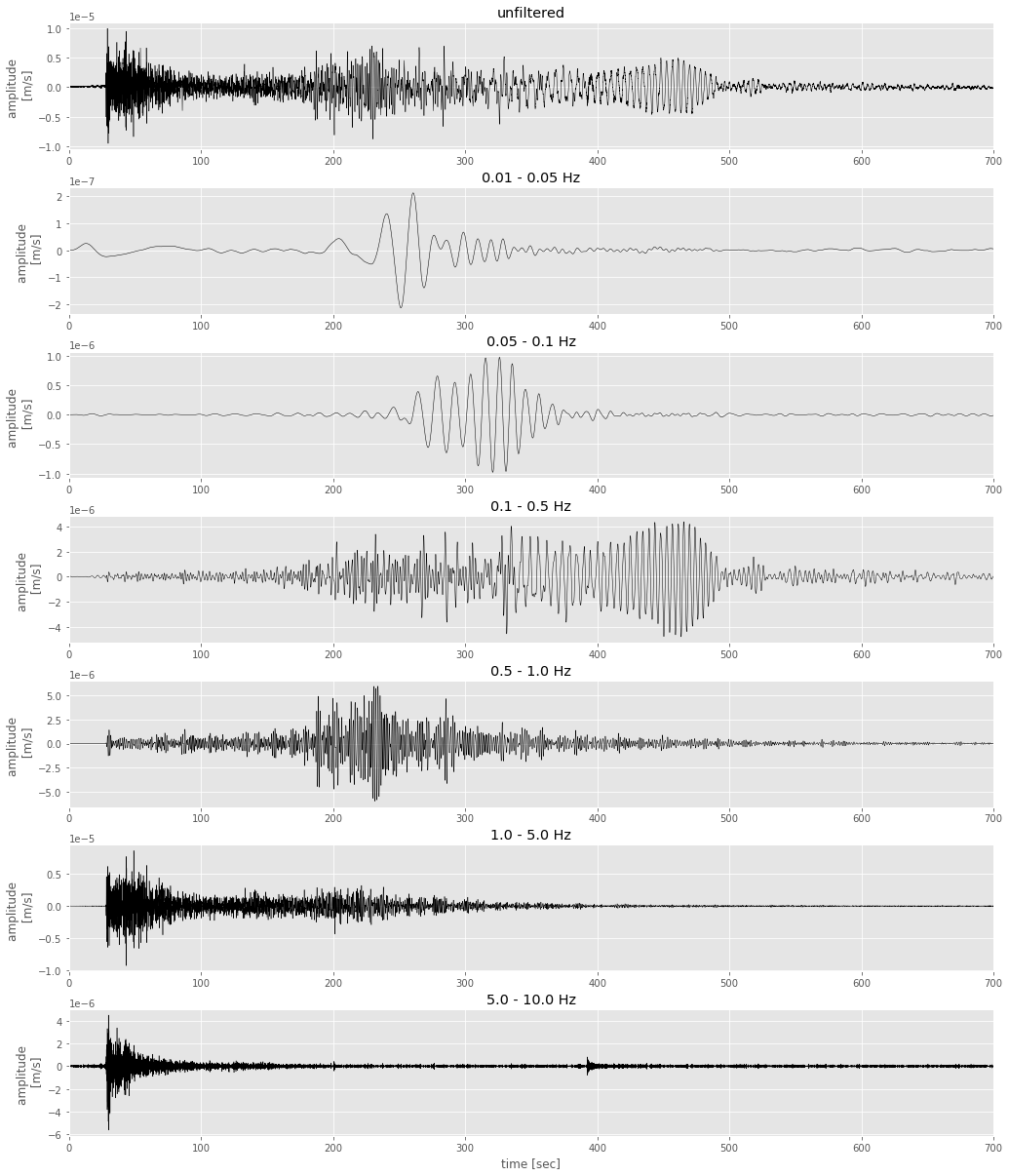Signal Processing
Filtering Basics - Solution

Seismo-Live: http://seismo-live.org

# Basics in filtering

In :
# Cell 0 - Preparation: load packages, set some basic options
%matplotlib inline
from obspy import *
from obspy.clients.fdsn import Client
import numpy as np
import matplotlib.pylab as plt
plt.style.use('ggplot')
plt.rcParams['figure.figsize'] = 15, 4
plt.rcParams['lines.linewidth'] = 0.5


## The filter¶

A good definition of what a filter is can be found in the book 'Of poles and zeros' by Frank Scherbaum:

Filters or systems are, in the most general sense, devices (in the physical world) or algorithms (in the mathematical world) which act on some input signal to produce a - possibly different - output signal

In seismology, filters are used to correct for the instrument response, avoid aliasing effects, separate 'wanted' from 'unwanted' frequencies, identify harmonic signals, model a specific recording instrument, and much more ...

There is no clear classification of filters. Roughly speaking, we can distinguish linear vs. non-linear, analog (circuits, resistors, conductors) vs. digital (logical components), and continuous vs. discrete filters. In seismology, we generally avoid non-linear filters, because their output contains frequencies which are not in the input signal. Analog filters can be continuous or discrete, while digital filters are always discrete. Discrete filters can be subdivided into infinite impulse response (IIR) filters, which are recursive and causal, and finite impulse response (FIR) filters, which are non-recursive and causal or acausal. We will explain more about these types of filters below.
Some filters have a special name, such as Butterworth, Chebyshev or Bessel filters, but they can also be integrated in the classification described above.

A filter is characterised by its frequency response function which is the Fourier transformation of the output signal divided by the Fourier transformation of the input signal:

$$T(j\omega) = \frac{Y(j\omega)}{X(j\omega)}$$

For a simple lowpass filter it is given as:

$$|T(j\omega)| = \sqrt{ \frac{1}{1+(\frac{\omega}{\omega_c})^{2n}} }$$

with $\omega$ indicating the frequency samples, $\omega_c$ the corner frequency of the filter, and $n$ the order of the filter (also called the number of corners of the filter). For a lowpass filter, all frequencies lower than the corner frequency are allowed to pass the filter. This is the pass band of the filter. On the other hand, the range of frequencies above the corner frequency is called stop band. In between lies the transition band, a small band of frequencies in which the passed amplitudes are gradually decreased to zero. The steepness of the slope of this transition band is defined by the order of the filter: the higher the order, the steeper the slope, the more effectively 'unwanted' frequencies get removed.

In the time domain, filtering means to convolve the data with the impulse response function of the filter. Doing this operation in the time domain is mathematically complex, computationally expensive and slow. Therefore, the digital application of filters is almost always done in the frequency domain, where it simplifies to a much faster multiplication between data and filter response. The procedure is as follows: transfer the signal into the frequency domain via FFT, multiply it with the filter's frequency response function (i.e. the FFT of the impulse response function), and transfer the result back to the time-domain. As a consequence, when filtering, we have to be aware of the characteristics and pit-falls of the Fourier transformation.

### Filter types¶

There are 4 main types of filters: a lowpass, a highpass, a bandpass, and a bandstop filter. Low- and highpass filters only have one corner frequency, allowing frequencies below and above this corner frequency to pass the filter, respectively. In contrast, bandpass and bandstop filters have two corner frequencies, defining a frequency band to pass and to stop, respectively.
Here, we want to see how exactly these filters act on the input signal. In Cell 1, the vertical component of the M$_w\,$9.1 Tohoku earthquake, recorded at Wettzell - Germany, is downloaded and pre-processed. In Cell 2, the four basic filters are applied to these data and plotted together with the filter functions and the resulting amplitude spectrum.

1) Look at the figure and explain what the different filters do.
2) Change the order of the filter (i.e the number of corners). What happens and why?

In :
# Cell 1: prepare data from Tohoku earthquake.
client = Client("BGR")
t1 = UTCDateTime("2011-03-11T05:00:00.000")
st = client.get_waveforms("GR", "WET", "", "BHZ", t1, t1 + 6 * 60 * 60,
attach_response = True)
st.remove_response(output="VEL")
st.detrend('linear')
st.detrend('demean')
st.plot()Out:In :
# Cell 2 - filter types
npts = st.stats.npts                   # number of samples in the trace
dt = st.stats.delta                    # sample interval
fNy = 1. / (2. * dt)                      # Nyquist frequency
time = np.arange(0, npts) * dt            # time axis for plotting
freq = np.linspace(0, fNy, npts // 2 + 1) # frequency axis for plotting
corners = 4                               # order of filter
# several filter frequencies for the different filter types
f0 = 0.04
fmin1 = 0.04
fmax1 = 0.07
fmin2 = 0.03
fmax2 = 0.07

# filter functions
LP = 1 / ( 1 + (freq / f0) ** (2 * corners))
HP = 1 - 1 / (1 + (freq / f0) ** (2 * corners))
wc = fmax1 - fmin1
wb = 0.5 * wc + fmin1
BP = 1/(1 + ((freq - wb) / wc) ** (2 * corners))
wc = fmax2 - fmin2
wb = 0.5 * wc + fmin2
BS = 1 - ( 1 / (1 + ((freq - wb) / wc) ** (2 * corners)))

# filtered traces
stHP = st.copy()
stHP.filter('highpass', freq=f0, corners=corners, zerophase=True)
stLP = st.copy()
stLP.filter('lowpass', freq=f0, corners=corners, zerophase=True)
stBP = st.copy()
stBP.filter('bandpass', freqmin=fmin1, freqmax=fmax1, corners=corners, zerophase=True)
stBS = st.copy()
stBS.filter('bandstop', freqmin=fmin2, freqmax=fmax2, corners=corners, zerophase=True)

# amplitude spectras
Ospec = np.fft.rfft(st.data)
LPspec = np.fft.rfft(stLP.data)
HPspec = np.fft.rfft(stHP.data)
BPspec = np.fft.rfft(stBP.data)
BSspec = np.fft.rfft(stBS.data)

# ---------------------------------------------------------------
# plot
plt.rcParams['figure.figsize'] = 17, 17
tx1 = 3000
tx2 = 8000
fx2 = 0.12

fig = plt.figure()

ax1.ticklabel_format(style='sci', axis='y', scilimits=(-1,1))
plt.plot(time, st.data, 'k')
plt.xlim(tx1, tx2)
plt.title('time-domain data')
plt.ylabel('original data \n amplitude [ms$^-1$]')

plt.plot(freq, abs(Ospec), 'k')
plt.title('frequency-domain data \n amplitude spectrum')
plt.ylabel('amplitude')
plt.xlim(0,fx2)

ax4.ticklabel_format(style='sci', axis='y', scilimits=(-1,1))
plt.plot(time, stLP.data, 'k')
plt.xlim(tx1, tx2)
plt.ylabel('LOWPASS  \n amplitude [ms$^-1$]')

plt.plot(freq, LP, 'k', linewidth=1.5)
plt.xlim(0,fx2)
plt.ylim(-0.1,1.1)
plt.title('filter function')
plt.ylabel('amplitude [%]')

plt.plot(freq, abs(LPspec), 'k')
plt.ylabel('amplitude ')
plt.xlim(0,fx2)

ax7.ticklabel_format(style='sci', axis='y', scilimits=(-1,1))
plt.plot(time, stHP.data, 'k')
plt.xlim(tx1, tx2)
plt.ylabel('HIGHPASS  \n amplitude [ms$^-1$]')

plt.plot(freq, HP, 'k', linewidth=1.5)
plt.xlim(0,fx2)
plt.ylim(-0.1,1.1)
plt.ylabel('amplitude [%]')

plt.plot(freq, abs(HPspec), 'k')
plt.ylabel('amplitude ')
plt.xlim(0,fx2)

ax10.ticklabel_format(style='sci', axis='y', scilimits=(-1,1))
plt.plot(time, stBP.data, 'k')
plt.xlim(tx1, tx2)
plt.ylabel('BANDPASS  \n amplitude [ms$^-1$]')

plt.plot(freq, BP, 'k', linewidth=1.5)
plt.xlim(0,fx2)
plt.ylim(-0.1,1.1)
plt.ylabel('amplitude [%]')

plt.plot(freq, abs(BPspec), 'k')
plt.ylabel('amplitude ')
plt.xlim(0,fx2)

ax13.ticklabel_format(style='sci', axis='y', scilimits=(-1,1))
plt.plot(time, stBS.data, 'k')
plt.xlim(tx1, tx2)
plt.xlabel('time [sec]')
plt.ylabel('BANDSTOPP \n amplitude [ms$^-1$]')

plt.plot(freq, BS, 'k', linewidth=1.5)
plt.xlim(0,fx2)
plt.ylim(-0.1,1.1)
plt.ylabel('amplitude [%]')
plt.xlabel('frequency [Hz]')

plt.plot(freq, abs(BSspec), 'k')
plt.xlabel('frequency [Hz]')
plt.ylabel('amplitude ')
plt.xlim(0,fx2)

plt.show()### Causal versus acausal¶

Filters can be causal or acausal. The output of a causal filter depends only on past and present input, while the output also depends on future input. Thus, an acausal filter is always symmetric and a causal one not. In this exercise, we want to see the effects of such filters on the signal. In Cell 3, the example seismogram of ObsPy is loaded and lowpass filtered several times with different filter order $n$ and causality.

3) Explain the effects of the different filters. You can also play with the order of the filter (variable $ncorners$).
4) Zoom into a small window around the first onset (change the variables $start$, $end$ and $amp$). Which filter would you use for which purpose?

In :
# Cell 3 - filter effects
tr = stf                    # select the first trace in the Stream object
tr.detrend('demean')           # preprocess data
tr.detrend('linear')
tr.filter("highpass", freq=2)  # removing long-period noise
print(tr)
t = tr.times()                 # time vector for x axis

f = 15.0                       # frequency for filters (intial: 10 Hz)
start = 4                      # start time to plot in sec (initial: 4)
end = 8                        # end time to plot in sec (initial: 8)
amp = 1500                     # amplitude range for plotting (initial: 1500)
ncorners = 4                   # number of corners/order of the filter (initial: 4)

tr_filt = tr.copy()            # causal filter / not zero phase. Order = 2
tr_filt.filter('lowpass', freq=f, zerophase=True, corners=2)
tr_filt2 = tr.copy()           # causal filter / not zero phase. Order = set by ncorners
tr_filt2.filter('lowpass', freq=f, zerophase=True, corners=ncorners)
tr_filt3 = tr.copy()           # acausal filter / zero phase. Order = set by ncorners
tr_filt3.filter('lowpass', freq=f, zerophase=False, corners=ncorners)

# plot - comment single lines to better see the remaining ones
plt.rcParams['figure.figsize'] = 15, 4
plt.plot(t, tr.data, 'k', label='original', linewidth=1.)
plt.plot(t, tr_filt.data, 'b', label='causal, n=2', linewidth=1.2)
plt.plot(t, tr_filt2.data, 'r', label='causal, n=%s' % ncorners, linewidth=1.2)
plt.plot(t, tr_filt3.data, 'g', label='acausal, n=%s' % ncorners, linewidth=1.2)

plt.xlabel('time [s]')
plt.xlim(start, end)
plt.ylim(-amp, amp)
plt.ylabel('amplitude [arbitrary]')
plt.legend(loc='lower right')

plt.show()

BW.RJOB..EHZ | 2009-08-24T00:20:03.000000Z - 2009-08-24T00:20:32.990000Z | 100.0 Hz, 3000 samples### Frequency ranges - bandpass filter¶

We will now look at an event which took place in Kazakhstan on July 8, 1989. We want to see how filtering helps to derive information from a signal. The signal has been recorded by a Chinese station and is bandpassed in several different frequency bands.

5) What do you see in the different frequency bands?
6) Play with the channel used for filtering in Cell 5. What do you not see? Can you guess what kind of event it is?

In :
# Cell 4 - get + preprocess data
c = Client("IRIS")
tmp1 = UTCDateTime("1989-07-08T03:40:00.0")
tmp2 = UTCDateTime("1989-07-08T04:05:00.0")
dat = c.get_waveforms("CD", "WMQ", "", "BH*", tmp1, tmp2, attach_response = True)
dat.detrend('linear')
dat.detrend('demean')
dat.remove_response(output="VEL")
dat.detrend('linear')
dat.detrend('demean')
print(dat)
#dat.plot()

3 Trace(s) in Stream:
CD.WMQ..BHE | 1989-07-08T03:48:37.790000Z - 1989-07-08T04:04:41.040000Z | 20.0 Hz, 19266 samples
CD.WMQ..BHN | 1989-07-08T03:48:37.790000Z - 1989-07-08T04:04:41.040000Z | 20.0 Hz, 19266 samples
CD.WMQ..BHZ | 1989-07-08T03:48:37.790000Z - 1989-07-08T04:04:41.040000Z | 20.0 Hz, 19266 samples

In :
# Cell 5 - filter data in different frequency ranges

chanel = 2
tm = dat[chanel].times()
xmin = 0
xmax = 700

dat1 = dat[chanel].copy()
dat2 = dat[chanel].copy()
dat2.filter(type="bandpass", freqmin=0.01, freqmax=0.05)
dat3 = dat[chanel].copy()
dat3.filter(type="bandpass", freqmin=0.05, freqmax=0.1)
dat4 = dat[chanel].copy()
dat4.filter(type="bandpass", freqmin=0.1, freqmax=0.5)
dat5 = dat[chanel].copy()
dat5.filter(type="bandpass", freqmin=0.5, freqmax=1)
dat6 = dat[chanel].copy()
dat6.filter(type="bandpass", freqmin=1., freqmax=5.)
dat7 = dat[chanel].copy()
dat7.filter(type="bandpass", freqmin=5., freqmax=10.)

plt.rcParams['figure.figsize'] = 17, 21
fig = plt.figure()
ax1.ticklabel_format(style='sci', axis='y', scilimits=(-1,1))
plt.plot(tm, dat1.data, 'k')
plt.xlim(xmin, xmax)
plt.title('unfiltered')
plt.ylabel('amplitude \n [m/s]')
ax2.ticklabel_format(style='sci', axis='y', scilimits=(-1,1))
plt.plot(tm, dat2.data, 'k')
plt.xlim(xmin, xmax)
plt.title('0.01 - 0.05 Hz')
plt.ylabel('amplitude \n [m/s]')
ax3.ticklabel_format(style='sci', axis='y', scilimits=(-1,1))
plt.plot(tm, dat3.data, 'k')
plt.xlim(xmin, xmax)
plt.title('0.05 - 0.1 Hz')
plt.ylabel('amplitude \n [m/s]')
ax4.ticklabel_format(style='sci', axis='y', scilimits=(-1,1))
plt.plot(tm, dat4.data, 'k')
plt.xlim(xmin, xmax)
plt.title('0.1 - 0.5 Hz')
plt.ylabel('amplitude \n [m/s]')
ax5.ticklabel_format(style='sci', axis='y', scilimits=(-1,1))
plt.plot(tm, dat5.data, 'k')
plt.xlim(xmin, xmax)
plt.title('0.5 - 1.0 Hz')
plt.ylabel('amplitude \n [m/s]')
ax6.ticklabel_format(style='sci', axis='y', scilimits=(-1,1))
plt.plot(tm, dat6.data, 'k')
plt.xlim(xmin, xmax)
plt.title('1.0 - 5.0 Hz')
plt.ylabel('amplitude \n [m/s]')
ax7.ticklabel_format(style='sci', axis='y', scilimits=(-1,1))
plt.plot(tm, dat7.data, 'k')
plt.xlim(xmin, xmax)
plt.title('5.0 - 10.0 Hz')
plt.xlabel('time [sec]')
plt.ylabel('amplitude \n [m/s]')
plt.show()

/Users/lion/miniconda3/envs/seismo_live/lib/python3.7/site-packages/obspy/signal/filter.py:67: UserWarning: Selected high corner frequency (10.0) of bandpass is at or above Nyquist (10.0). Applying a high-pass instead.
warnings.warn(msg)